STMY

•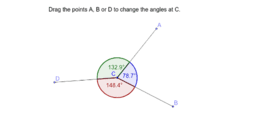Angles at a Point

Activity

STMY

•Parallelogram

Activity

STMY

•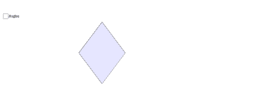Rhombus

Activity

STMY

•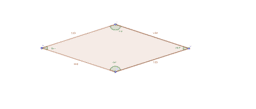Activity

STMY

•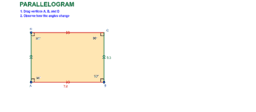Angles in a Parallelogram

Activity

STMY

•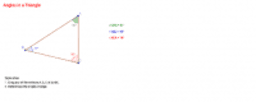Angles in a Triangle

Activity

STMY

•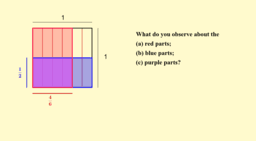Multiplication of Proper Fractions

Activity

STMY

•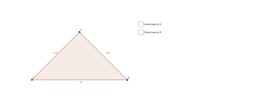Area of Triangle (Challenge) - Base

Activity

STMY

•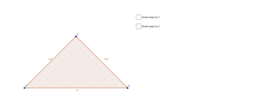Area of Triangle (Challenge) - Angle

Activity

STMY

•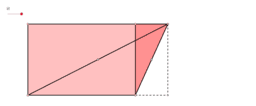Area of Triangle 2

Activity

STMY

•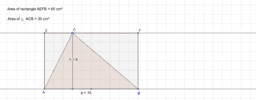Area of Triangle

Activity

STMY

•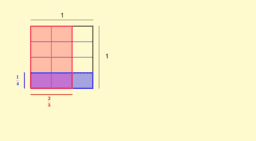Multiplication of Fractions

Activity

STMY

•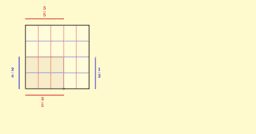Multiplication of Fractions

Activity

STMY

•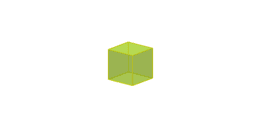Cube

Activity

STMY

•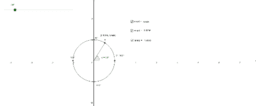Trigonometry on the Circle

Activity

STMY

•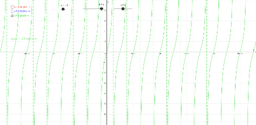Transformation of Trigonometric Graphs

Activity

STMY

•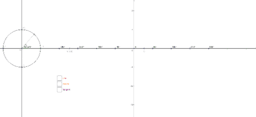Basic Trigonometric Graphs

Activity

STMY

•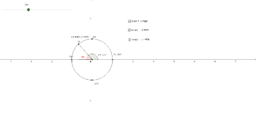Activity

STMY